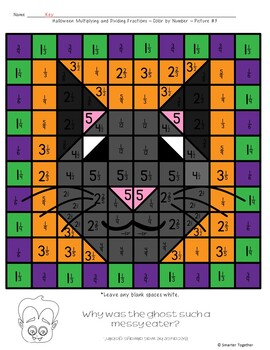# Multiplying and Dividing Fractions Halloween ActivitySubject
Resource Type
File Type

PDF

(3 MB|6 student pages)
Product Rating
Standards
• Product Description
• StandardsNEW

Make multiplying and dividing fractions fun when students use products and quotients to color Halloween themed pictures. Includes 3 leveled activity sheets (multiplication, division, and a mixed review). Each activity set includes 20 problems and a corresponding color by code sheet.

Picture #1 - Multiplication of fractions by whole numbers, fractions, and mixed numbers

Picture #2 - Division of fractions by whole numbers and whole numbers by fractions

Picture #3 - Mixed Review - Multiply and divide fractions

Perfect for math centers, homework, or leave with a substitute! Easy to use and engaging while still maintaining a strong academic focus.

Looking for a version you can use year round? Check out Multiplying and Dividing Fractions Color By Number Activity here!

Interpret division of a whole number by a unit fraction, and compute such quotients. For example, create a story context for 4 ÷ (1/5), and use a visual fraction model to show the quotient. Use the relationship between multiplication and division to explain that 4 ÷ (1/5) = 20 because 20 × (1/5) = 4.
Interpret division of a unit fraction by a non-zero whole number, and compute such quotients. For example, create a story context for (1/3) ÷ 4, and use a visual fraction model to show the quotient. Use the relationship between multiplication and division to explain that (1/3) ÷ 4 = 1/12 because (1/12) × 4 = 1/3.
Apply and extend previous understandings of division to divide unit fractions by whole numbers and whole numbers by unit fractions.
Apply and extend previous understandings of multiplication to multiply a fraction or whole number by a fraction.
Total Pages
6 student pages
Included
Teaching Duration
N/A
Report this Resource to TpT
Reported resources will be reviewed by our team. Report this resource to let us know if this resource violates TpT’s content guidelines.